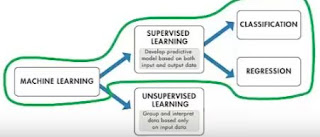# New Technology

Trending Technology Machine Learning, Artificial Intelligent, Block Chain, IoT, DevOps, Data Science

## Search This Blog

The Iris flower data set or Fisher's Iris data (also called Anderson's Iris data set) set is a multivariate data set introduced by the British statistician and biologist Ronald Fisher in his 1936 paper "The use of multiple measurements in taxonomic problems".

The iris dataset contains the following data:
The format for the data:
(sepal length, sepal width, petal length, petal width, Category)

Step 01
Get the data.

Step 02
Prepare the data.
Clean data, combine datasets, and prepare it for analysis.

Step 03
Train the model
Feed the information into the machine to teach it what to expect.

Step 04
Predict future demand
Use the model to forecast future spikes and shortfalls in demand.

Step 05
Score and evaluate the model
Test the model's ability to predict the original data, and evaluate its success.

UCI Machine Learning Repository
• The UCI Machine Learning Repository is a collection of databases that are used by the machine learning community for the empirical analysis of machine learning algorithms. It has been widely used by students, educators, and researches all over the world as a primary source of machine learning data sets.
Kaggle
• Kaggle is a platform for predictive modelling and data science competitions.
The libraries which we will be needed are:
• Numpy
• Scipy
• Pandas
• Matplotlib
• Scikit-learn

Iris Datasets Prediction - Steps1. Identification of problem.
- Supervised learning problem
* Regression
* Classification

2. Identification of different variables/features in data i.e textual, numerical, categorical etc.

First 50 samples are Iris setosa Second 50 sample are Iris virginica, and Third and Last 50 samples are Iris versicolor.

3. Convert the textual and categorical variables in to numerical in order to make the data into a suitable format so that machine learning can be applied to it.
- Machine learning algorithms can directly apply to numerical variables.

4. Splitting the data into different parts i.e. training set and testing set.
- Training Set is a dataset of examples used to fill the parameters of the model. The model is trained on the training dataset using a supervised or unsupervised learning method. Hence the model is initially fit on a training dataset.
- Test Set is a dataset used to provide an unbiased evaluation of a final model fit on the training dataset.

5. Now the major step is model selection. We generally use the following algorithms in the process of selecting a machine learning model:
- Regression
- Classification

6. Evaluation metric to evaluate the result or accuracy of our model. For any machine learning problem there are numourious evaluation matrices. Separate for classification and regression.
- Root Mean Squared Error
- Precision & Recall
- Accuracy
- Logarithmic Loss (log-loss)
- Confusion Matrix
- Gain and Lift Chart
- AUC-ROC (Area Under the Receiver Operating Characteristic Curve)
- Gini Coefficient

1.nice information on data science has given thank you very much.
2.3.